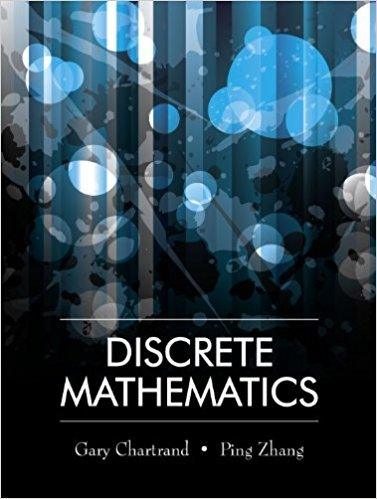×
×

# Solutions for Chapter 2.1: Sets and Subsets## Full solutions for Discrete Mathematics | 1st Edition

ISBN: 9781577667308Solutions for Chapter 2.1: Sets and Subsets

Solutions for Chapter 2.1
4 5 0 360 Reviews
14
0
##### ISBN: 9781577667308

This expansive textbook survival guide covers the following chapters and their solutions. Since 26 problems in chapter 2.1: Sets and Subsets have been answered, more than 13675 students have viewed full step-by-step solutions from this chapter. Discrete Mathematics was written by and is associated to the ISBN: 9781577667308. This textbook survival guide was created for the textbook: Discrete Mathematics, edition: 1. Chapter 2.1: Sets and Subsets includes 26 full step-by-step solutions.

Key Math Terms and definitions covered in this textbook
• Affine transformation

Tv = Av + Vo = linear transformation plus shift.

• Basis for V.

Independent vectors VI, ... , v d whose linear combinations give each vector in V as v = CIVI + ... + CdVd. V has many bases, each basis gives unique c's. A vector space has many bases!

• Hilbert matrix hilb(n).

Entries HU = 1/(i + j -1) = Jd X i- 1 xj-1dx. Positive definite but extremely small Amin and large condition number: H is ill-conditioned.

• Identity matrix I (or In).

Diagonal entries = 1, off-diagonal entries = 0.

• Indefinite matrix.

A symmetric matrix with eigenvalues of both signs (+ and - ).

• Inverse matrix A-I.

Square matrix with A-I A = I and AA-l = I. No inverse if det A = 0 and rank(A) < n and Ax = 0 for a nonzero vector x. The inverses of AB and AT are B-1 A-I and (A-I)T. Cofactor formula (A-l)ij = Cji! detA.

• Kronecker product (tensor product) A ® B.

Blocks aij B, eigenvalues Ap(A)Aq(B).

• Multiplication Ax

= Xl (column 1) + ... + xn(column n) = combination of columns.

• Multiplier eij.

The pivot row j is multiplied by eij and subtracted from row i to eliminate the i, j entry: eij = (entry to eliminate) / (jth pivot).

• Particular solution x p.

Any solution to Ax = b; often x p has free variables = o.

• Pivot.

The diagonal entry (first nonzero) at the time when a row is used in elimination.

• Polar decomposition A = Q H.

Orthogonal Q times positive (semi)definite H.

• Projection matrix P onto subspace S.

Projection p = P b is the closest point to b in S, error e = b - Pb is perpendicularto S. p 2 = P = pT, eigenvalues are 1 or 0, eigenvectors are in S or S...L. If columns of A = basis for S then P = A (AT A) -1 AT.

• Projection p = a(aTblaTa) onto the line through a.

P = aaT laTa has rank l.

• Random matrix rand(n) or randn(n).

MATLAB creates a matrix with random entries, uniformly distributed on [0 1] for rand and standard normal distribution for randn.

• Row picture of Ax = b.

Each equation gives a plane in Rn; the planes intersect at x.

• Saddle point of I(x}, ... ,xn ).

A point where the first derivatives of I are zero and the second derivative matrix (a2 II aXi ax j = Hessian matrix) is indefinite.

• Schur complement S, D - C A -} B.

Appears in block elimination on [~ g ].

• Stiffness matrix

If x gives the movements of the nodes, K x gives the internal forces. K = ATe A where C has spring constants from Hooke's Law and Ax = stretching.

• Subspace S of V.

Any vector space inside V, including V and Z = {zero vector only}.

×